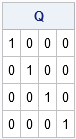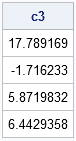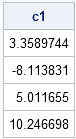In a previous post, I discussed computing regression coefficients in different polynomial bases and showed how the coefficients change when you change the basis functions. In particular, I showed how to convert the coefficients computed in one basis to coefficients computed with respect to a different basis.

It turns out that there is one set of polynomial basis functions for which the regression coefficients are particularly easy to compute. They are called orthogonal polynomials, and you can compute them in SAS/IML software by using the ORPOL function. The design matrix that is returned by the ORPOL function is orthonormal, which means that each column of the matrix is orthogonal to every other column and is standardized to have unit standard deviation.

### Orthogonal Polynomials

Given a set of points, x, you can evaluate the orthogonal polynomials at x by using the following statements:

```proc iml; x = T(do(-1, 1, 0.2)); B3 = orpol(x, 3); /** design matrix of orthogonal polynomials **/```

You can verify that the columns are orthonormal by computing the matrix of crossproducts:

```Q = B3` * B3; /** verify B3` * B3 = I **/ reset fuzz; /** print tiny numbers as zeros **/ print Q;```This result is important because it means that the normal equations for polynomial regression are greatly simplified when you use the B3 basis. In general, you can compute the regression coefficients for any design matrix by using the normal equations. However, because the columns of B3 are orthonormal, the first term in the normal equations is simply the identity matrix! Therefore, the normal equations simplify, as shown in the following statements:

```y = {6,8,8,7,5,3,2,1,3,6,10}; /** compute parameter estimates by solving normal equations **/ /** c3 = inv(B3` * B3) * (B3` * y); **/ /** = I * (B3` * y); **/ c3 = B3` * y; /** parameter estimates (orthogonormal data matrix) **/ print c3;```Can it really be so simple? Yes. By using the orthogonal basis functions from the ORPOL function, linear regression simplifies to a single matrix multiplication.

### Interpreting the Coefficients by Changing Bases

The regression coefficients computed in the basis of orthogonal polynomials are not easy to interpret, so you might be interested in converting them to the standard basis of monomials, (1, x, x2, x3).

You could try to write down the transition matrix, as used in my previous post, but it is not trivial to write the orthogonal polynomials used to construct the B3 matrix in terms of the standard monomials. Instead, you can use a trick suggested by one of my SAS colleagues who noted that the predicted values for the orthogonal basis are the same as the predicted valued in any other basis. (The predicted values are obtained by the formula p = B3 * c1.) That means that if c1 are the regression coefficients computed in the standard monomial basis and c3 are the regression coefficients computed in the basis of orthogonal polynomials, then you can equate the predicted values:

``````
B1 * c1 = B3 * c3
``````

Because B3 is orthonormal, multiply both sides by the transpose of B3, which simplifies the right-hand side:

``````
B3` * B1 * c1 = c3
``````

This leads to the following SAS/IML statements that compute the regression coefficients with respect to the standard monomial basis in terms of the c3 coefficients that were computed previously:

```/** define standard polynomial basis **/ Intercept = j(nrow(x), 1, 1); B1 = Intercept || x || x##2 || x##3; c1 = inv(B3` * B1) * c3; /** compute standard regression coefficients **/ print c1;```Sure enough, if you look back at my previous post, you will see that these are regression coefficients for the data in the standard polynomial basis.

ShareDistinguished Researcher in Computational Statistics

Rick Wicklin, PhD, is a distinguished researcher in computational statistics at SAS and is a principal developer of SAS/IML software. His areas of expertise include computational statistics, simulation, statistical graphics, and modern methods in statistical data analysis. Rick is author of the books Statistical Programming with SAS/IML Software and Simulating Data with SAS.

1.•